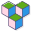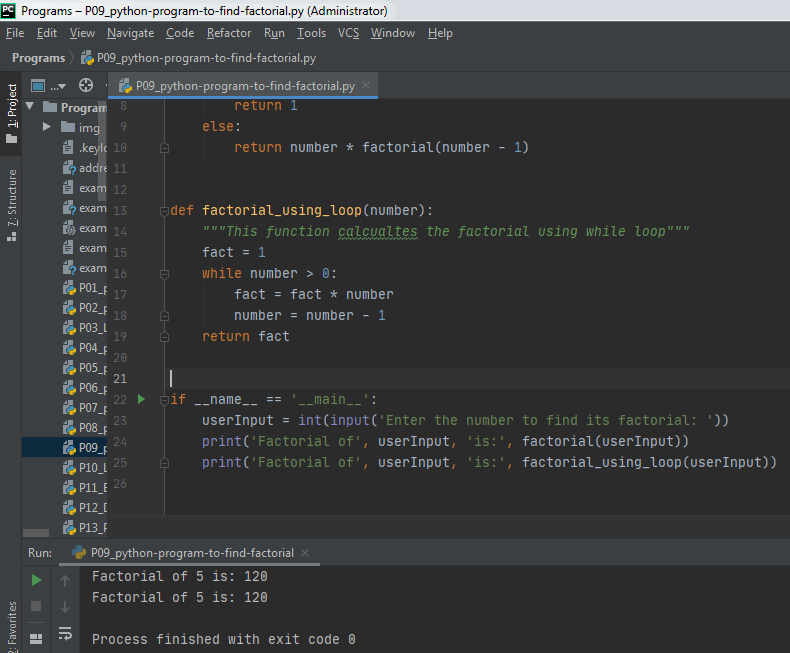CppBuzz# Python program to find factorial

# Author: CppBuzz.com
# This program calculates the factorial of a given number using recursion & normal method
# Date: 22nd Aug 2020

```
def factorial(number):
"""This function calculates the factorial of any number"""
if number == 1 or number == 0:
return 1
else:
return number * factorial(number - 1)

def factorial_using_loop(number):
"""This function calculates the factorial using while loop"""
fact = 1
while number > 0:
fact = fact * number
number = number - 1
return fact

if __name__ == '__main__':
userInput = int(input('Enter the number to find its factorial: '))
print('Factorial of', userInput, 'is:', factorial(userInput))
print('Factorial of', userInput, 'is:', factorial_using_loop(userInput))

```